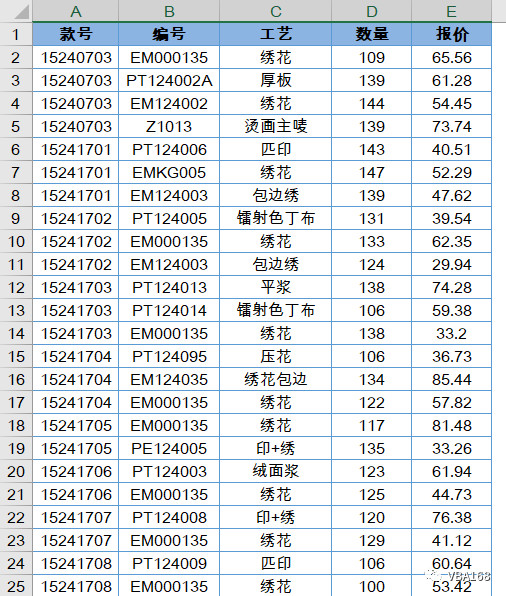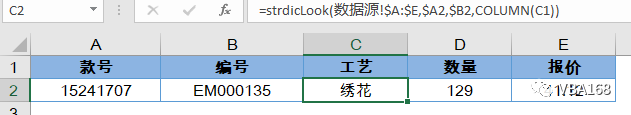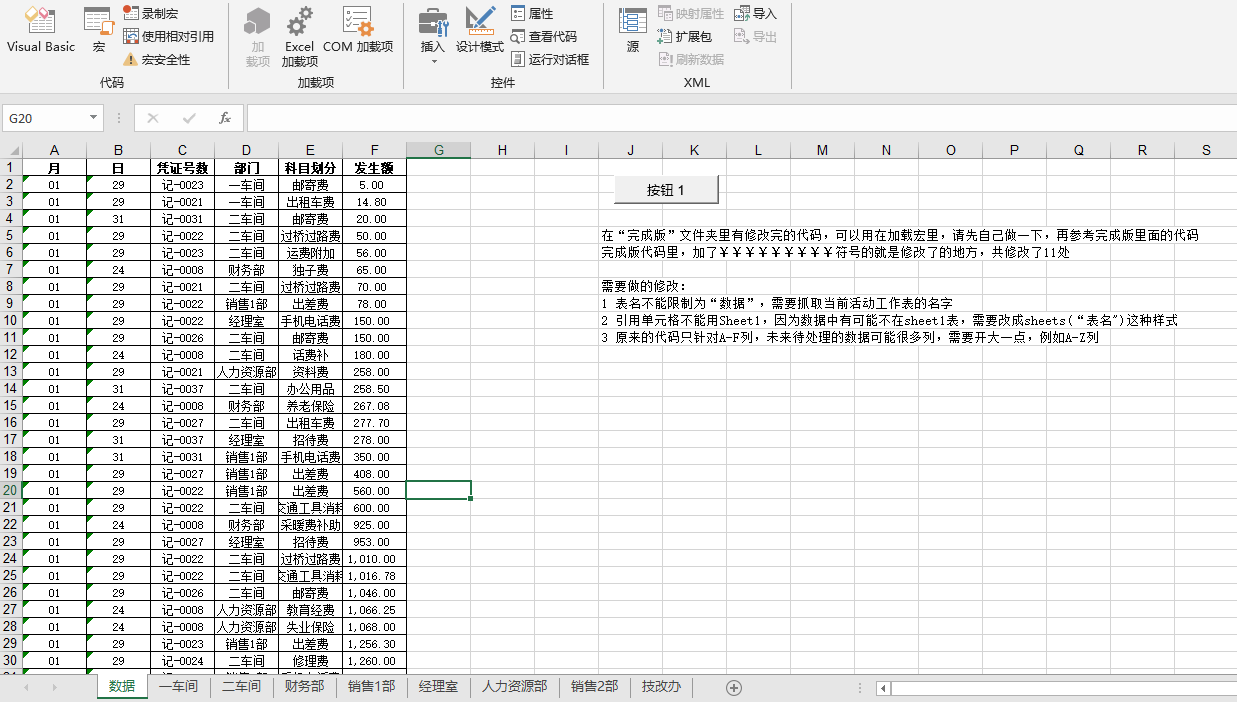• VBA实现EXCEL表格多条件查询 For i = 1 To Workbooks.Count For Each c In Sheets(i).UsedRange If c.Value >= 150 And c.Value c1 = c1 + c.Value n = n + 1 End If ‘更多条件…… Next Next
• ## Excel VBA多条件查询

万次阅读 2013-08-26 15:19:12
用以下两个公式都可以实现多条件查询，同样也可以用VBA代码来实现 函数公式1： '=INDEX(Sheet3!$F$2:$F$20,MATCH(B2&C2&D2&E2,Sheet3!$B$2:$B$20&Sheet3!$C$2:$C$20&Sheet3!$D$2:$D$20&Sheet3!$E$2:$E$20,0)) 函数...
用以下两个公式都可以实现多条件查询，同样也可以用VBA代码来实现
函数公式1： '=INDEX(Sheet3!$F$2:$F$20,MATCH(B2&C2&D2&E2,Sheet3!$B$2:$B$20&Sheet3!$C$2:$C$20&Sheet3!$D$2:$D$20&Sheet3!$E$2:$E$20,0))
函数公式2： '=LOOKUP(1,0/(B3&C3&D3&E3=stock!$B$2:$B$20&stock!$C$2:$C$20&stock!$D$2:$D$20&stock!$E$2:$E$20),stock!$F$2:$F$20)

Sub 多条件查询() Dim arr, brr, d, s$, i&, t$ Set d = CreateObject("scripting.dictionary") '创建字典 arr = Sheets(2).Range("b2", Sheets(2).[h65536].End(3)).Value '将所要查询的区域定义到数组arr中  For i = 1 To UBound(arr)     s = Join(Application.Index(Application.Index(arr, i, 0), Array(1, 2, 3, 4)), "|") '将数组arr每行的四个元素通过"|"拼接成为一个字符串     d(s) = arr(i, 6) '数组arr的第6列元素存入字典  Next brr = Sheets(4).Range("b2", Sheets(4).[h65536].End(3)).Value '当前要查询的target区域定义到数组brr中  For i = 1 To UBound(brr)     t = Join(Application.Index(Application.Index(brr, i, 0), Array(1, 2, 3, 4)), "|")     If d.exists(t) Then brr(i, 5) = d(t) Else brr(i, 5) = 0  Next Sheets(4).[b2].Resize(UBound(brr), UBound(brr, 2)) = brr '重新定义数组区域，并将brr重新赋值给它 Set d = Nothing
End Sub
展开全文• VBA示例函数之 求人不如自已动手 个工作表中查找一个范围内符合某个指定条件的项目对应指定范围加总求和 ，供初学者参考，大牛勿进~~~~~~~
• 多条件查询并返回列数据，是在数据查询时经常会使用的功能，利用字典与数组结合，能够高效地实现此功能。数据源图如下。 现需要根据款号，编号两个条件查询工艺、数量、报价信息，示例代码如下。 Function ...
多条件查询并返回多列数据，是在数据查询时经常会使用的功能，利用字典与数组结合，能够高效地实现此功能。数据源图如下。现需要根据款号，编号两个条件，查询工艺、数量、报价信息，示例代码如下。
Function strDicLook(ByVal rngRegion As Range _
, ByVal rngConditionO As Range _
, ByVal rngConditionT As Range _
, ByVal intColumn As Integer)AsString
Dim dicData As Object, avntList() As Variant
Dim i As Integer
Set dicData = CreateObject("scripting.dictionary")
avntList = Intersect(rngRegion, rngRegion.Parent.UsedRange).Value
For i = 1 To UBound(avntList)
dicData(avntList(i, 1) & avntList(i, 2)) = Array(avntList(i, 1), avntList(i, 2), avntList(i, 3), avntList(i, 4), avntList(i, 5))
Next i
strDicLook = dicData(rngConditionO & rngConditionT)(intColumn - 1)
End Function
第1行代码声明4个参数的自定义函数，其返回值为字符串类型。第1个参数指定查询的数据源区域，第2个参数和第3个参数分别指定查询的条件，第4个参数指定查询结果在数据源中的列数。
第10行代码将数组中前两列的数据合并作为字典的关键字，并将由Array函数创建的5个元素的一维数组写入该关键字对应的Item条目。
第12行代码读取该关键字对应的Item条目中的数组，并获取数组指定位置的元素。
由于数组不能根据指定的关键字直接检索数据，所以先在字典的Item中保存数组，再利用字典的关键字来查询对应的数据，这样可以极大地提升查询效率。
在单元格中使用StrDicLook函数，结果如下图所示。微信公众号：VBA168
淘宝店铺地址：https://item.taobao.com/item.htm?spm=a1z10.1-c-s.w4004-21233576391.4.1af0683dzrx3oU&id=584940166162
关注微信公众号，每天及时接收Excel VBA经典示例讲解。
淘宝店铺提供Excel定制服务。
祝你工作和学习更轻松！
展开全文• 利用VBA字典来实现多条件分类汇总, 重复值累加, 不重复值保存, 比普通的遍历数组比较, 更加简化代码, 易于理解.
• 字典列分类汇总,用VBA字典数组汇总多列,字典分组汇总的写法
• 如何使用VBA按月份及商品名称汇总其对应商品的销售数量和收入合计？ 日期 商品代码 商品名 数量 收入 2011/5/30 01.0014 ASCERT -800 -369231 2011/5/31 01.0014 ASCERT -...
目录
示例
代码

示例
如图所示，该表为某公司的销售数据。如何使用VBA按月份及商品名称汇总其对应商品的销售数量和收入合计？
日期商品代码商品名数量收入2011/5/3001.0014ASCERT-800-3692312011/5/3101.0014ASCERT-200-92307.72011/8/1701.0032ASWDVBN0.054273.52011/1/2801.0003ERTGH13589.742011/1/2901.0003ERTGH13846.152011/2/101.0004EFGTYUI113247.862011/2/201.0004EFGTYUI116239.322011/2/501.0007DFRTYHB11282.052011/1/2701.0003ERTGH311025.642011/4/2601.0012ZXSC3569.232011/4/2501.0011RGHUO871794.872011/4/2401.0011RGHUO1085470.092011/4/2801.0014ASCERT2511965.812011/5/2101.0014ASCERT2511858.972011/9/1101.0014ASCERT2511858.972011/2/301.0005WERDS40153846.22011/8/401.0028VGRYUI40102564.12011/10/1801.0014ASCERT5023717.952011/7/901.0014ASCERT5023717.952011/1/3101.0003ERTGH60256410.32011/1/3001.0003ERTGH74316239.32011/6/2501.0014ASCERT7535576.922011/2/1101.0007DFRTYHB1001085472011/2/1201.0007DFRTYHB100109401.7
代码
Option Explicit

Sub 按多列汇总数据()
Dim shtData As Worksheet
Dim shtSum As Worksheet
Dim rowSum As Long
Dim rowData As Long
Dim i As Long
Dim lMonth As Long
Dim sProd As String
Dim bln As Boolean

Set shtData = Sheets("sheet1")
Set shtSum = Sheets("sheet2")

rowSum = 2
shtSum.Range("A2:D" & Rows.Count).Clear

'获取汇总字段（月份，商品名称）的记录
For rowData = 2 To shtData.Range("A1").CurrentRegion.Rows.Count
lMonth = Month(shtData.Cells(rowData, "A").Value)
sProd = shtData.Cells(rowData, "C").Value
bln = True
For i = 2 To rowSum
If lMonth = shtSum.Cells(i, 1).Value And sProd = shtSum.Cells(i, 2).Value Then
bln = False
Exit For
End If
Next i
If bln Then
shtSum.Cells(rowSum, 1).Value = lMonth
shtSum.Cells(rowSum, 2).Value = sProd
rowSum = rowSum + 1
End If
Next rowData

'汇总
For rowSum = 2 To shtSum.Range("A1").CurrentRegion.Rows.Count
lMonth = shtSum.Cells(rowSum, "A").Value
sProd = shtSum.Cells(rowSum, "B").Value
For rowData = 2 To shtData.Range("A1").CurrentRegion.Rows.Count
If shtData.Cells(rowData, "C").Value = sProd And Month(shtData.Cells(rowData, "A").Value) = lMonth Then
shtSum.Cells(rowSum, "C").Value = shtSum.Cells(rowSum, "C").Value + shtData.Cells(rowData, "D").Value
shtSum.Cells(rowSum, "D").Value = shtSum.Cells(rowSum, "D").Value + shtData.Cells(rowData, "D").Value
End If
Next rowData
Next rowSum
End Sub



展开全文• 原有的Range(“a1:f” & irow)已经改为Range(“a1:z” & irow) 因为数据可能会列数很，所以写成到z列，故意写一些。￥￥￥￥￥￥￥￥￥￥￥￥￥ For j = 2 To Sheets.Count Sheets(str).Range(“a1:z” & irow)....
在一个工作簿中,想对某一列做筛选,并且拆分到后面的工作表里,通用代码如下: Sub chaifenshuju()
Dim sht As Worksheet Dim k, i, j As Integer Dim irow As Integer '这个说的是一共多少行 Dim l As Integer Dim sht0 As Worksheet
Set sht0 = ActiveSheet
l = InputBox(“请输入你要按哪列分”)
'删除无意义的表 Application.DisplayAlerts = False If Sheets.Count > 1 Then For Each sht1 In Sheets If sht1.Name <> sht0.Name Then sht1.Delete End If Next End If Application.DisplayAlerts = True '这个地方上课的时候我没改成true，请大家注意一下
irow = sht0.Range(“a65536”).End(xlUp).Row '拆分表 For i = 2 To irow k = 0 For Each sht In Sheets If sht.Name = sht0.Cells(i, l) Then k = 1 End If Next
If k = 0 Then
Sheets(Sheets.Count).Name = sht0.Cells(i, l)
End If

Next '拷贝数据
For j = 2 To Sheets.Count sht0.Range(“a1:z” & irow).AutoFilter Field:=l, Criteria1:=Sheets(j).Name sht0.Range(“a1:z” & irow).Copy Sheets(j).Range(“a1”) Next
sht0.Range(“a1:z” & irow).AutoFilter
sht0.Select
MsgBox “已处理完毕，牛逼不”
End SubSub chaifenshuju()
Dim sht As Worksheet Dim k, i, j As Integer Dim irow As Integer '这个说的是一共多少行 Dim l As Integer Dim str As String '这里是第1处修改，加入一个变量，用于提取当前工作表的名字￥￥￥￥￥￥￥￥￥￥￥￥￥￥￥
str = ActiveSheet.Name '这里是第2处修改，取得当前要处理的表的名字￥￥￥￥￥￥￥￥￥￥￥￥
l = InputBox(“请输入你要按哪列分”)
'删除无意义的表 Application.DisplayAlerts = False If Sheets.Count > 1 Then For Each sht1 In Sheets If sht1.Name <> str Then '这里是第3处修改，不在用“数据”这个固定的名字了，而是用之前取到的名字￥￥￥￥￥￥￥￥￥￥￥ sht1.Delete End If Next End If Application.DisplayAlerts = True '这个地方上课的时候我没改成true，请大家注意一下
irow = Sheets(str).Range(“a65536”).End(xlUp).Row '这里是第4处修改，不在用“sheet1”这个固定的表名字了，而是用之前取到的名字的表￥￥￥￥￥￥￥￥￥￥￥ '拆分表 For i = 2 To irow k = 0 For Each sht In Sheets If sht.Name = Sheets(str).Cells(i, l) Then '这里是第5处修改，跟第四个修改一样，把原来的sheet1替换成sheets(str)￥￥￥￥￥￥￥￥￥￥￥ k = 1 End If Next
If k = 0 Then
Sheets(Sheets.Count).Name = Sheets(str).Cells(i, l)   '这里是第6处修改，跟第四个修改一样，把原来的sheet1替换成sheets(str)￥￥￥￥￥￥￥￥￥￥￥
End If

Next '拷贝数据 注意：第7处修改。原有的Range(“a1:f” & irow)已经改为Range(“a1:z” & irow) 因为数据可能会列数很多，所以写成到z列，故意多写一些。￥￥￥￥￥￥￥￥￥￥￥￥￥
For j = 2 To Sheets.Count Sheets(str).Range(“a1:z” & irow).AutoFilter Field:=l, Criteria1:=Sheets(j).Name '这里是第8处修改，跟第四个修改一样，把原来的sheet1替换成sheets(str)￥￥￥￥￥￥￥￥￥￥￥ Sheets(str).Range(“a1:z” & irow).Copy Sheets(j).Range(“a1”) '这里是第9处修改，跟第四个修改一样，把原来的sheet1替换成sheets(str)￥￥￥￥￥￥￥￥￥￥￥ Next
Sheets(str).Range(“a1:z” & irow).AutoFilter '这里是第10处修改，跟第四个修改一样，把原来的sheet1替换成sheets(str)￥￥￥￥￥￥￥￥￥￥￥
Sheets(str).Select '这里是第11处修改，跟第四个修改一样，把原来的sheet1替换成sheets(str)￥￥￥￥￥￥￥￥￥￥￥
MsgBox “已处理完毕，牛逼不”
End Sub
展开全文• 不需要了解vba、公式，支持插入文件名、sheet名列，根据汉字生成汇总列 使用时自动提示 工具地址 ：http://www.excelfb.com/找到下图的工具 Excel自动汇总工具（根据汉字描述的要求格式进行汇总） ...
• 一个在Excel中使用VBA将所有sheet中的数据和sheet信息汇总到总表中的例子，使用VBA编写，在第一个sheet中的宏check，使用时需要打开excel的宏安全，执行时会在合计页填充所有其他sheet的sheet名称，链接，编号，合计...
• 在Excel中使用VBA创建脚本，以分析2014年至2016年的库存数据。 过程： 用于for loops和conditionals循环访问工作簿中3个工作表中的每一行数据，以返回以下内容： 股票代号 每个股票代号出售的股票总数 每个股票...
•  用户需求：媳妇统计excel时需要在个sheet页中搜索关键字，找到匹配的行后再粘贴到新的sheet页中，然后问我有什么快捷的方法，一键式的。 我想了想写个宏，碎碎个事。好了，开始！ 实现思路：打开excel，新建一...excel关键字匹配 sheet
• 因为用函数公式，如果数据量大的话，会影响效率，而且会增加文件本身大小，所以想用vba代码替代。 以下代码用来实现函数公式sumproduct的功能，实现多条件求和。 Private Sub 多条件求和()  Dim d, ar, arr, s$, t... • Option Explicit Sub test() Dim sht As Worksheet, rng As Range Set sht = ActiveSheet Dim i As Integer, index As Integer, subtotal As Double, dm As Variant Dim oldIndex As Integer, oldS... • '统计个excel表格的数据，每个行列值累加汇总到一个表格中 Sub huizong() '存放文件的目录 dirPath = "C:\Users\zhaigx\Desktop\1" fname = Dir(dirPath &amp; "\" &amp; "*....excel • 现希望建立一个汇总表，按月份和商品名汇总收入，并将月份作为列标题而商品名作为行标题。该如何用VBA实现？ 日期 商品代码 商品名 数量 收入 2011/8/17 01.0032 ASWDVBN 0.05 4273.5 ... • 看似没用的东西，在真正需要的时候就...'Union (AlL) 个select查询结果合并在一起 Sub 合并工作表数据() Dim data As New 类1 Dim sql As String sql = "select * from [Sheet1$a:c] union all select * fr...
• '''''''''''''''''''''''''''''''''''''''''''''筛选条件1  If Left(arr(i, 7), 6) = "mobile" And (arr(i, 8) = "A" Or arr(i, 8) = "B" Or arr(i, 8) = "C" Or arr(i, 8) = "D") Then    z = arr(i, 2) ...
• 实例 数据分类汇总 目标 ... 根据筛选条件逐一汇总到目标sheet move sheet 到新的工作簿 文件 不知怎们上传demo文件知道的留言一下 技术思辨 数据copy时候因为...
• If Cells(i, j).Value = DataName Then '更精准可以用Instr，条件，或者 用正则表达式 If Cells(i, j).MergeCells = True Then ReTurnRowNum = i Exit Do Exit For Exit Do Else: ReTurnRowNum = 0 End ...
• 心得(一)：关于VBA如何把同一个文件下的所有文件的内容合并到同一个excel文件下 问题：如何把个格式相同的excel整合到一个excel上，起初想的是可以直接用复制粘贴……但是文件有几百个将近一千个，这么做的话有点...
• 本人想从个EXCLE数据表中查询数据结果，想用EXCLE公式或者VBA实现，但是技术入门才，请教各位技术人才，帮写下 1.数据表EXCLE个（这里做了3个，实际可能成百上千），表名称规范统一命名的（和汇总表数据里名称列...windows
• ## Excel VBA 多重筛选

千次阅读 2019-08-13 02:40:29
Excel VBA 多重筛选 Sub FilterOnValues() 'PURPOSE: Filter on specific values Dim rng As Range Set rng = ActiveSheet.Range("B7:D18") FilterField = WorksheetFunction.Match("Country", rng.Rows(1), 0) ...
• 分表汇总 代码 Public Sub fenlei() Dim i, a, b, c, d, e As Integer Dim arr a = 2 b = 2 c = 2 d = 2 e = 2 row_num = Cells(Rows.Count, 3).End(xlUp).Row arr = Range("b3:i3") Worksheets.Add.Name = ...数据分析
• 将总数据表进行拆分至个工作表问题使用For循环拆分工作表使用筛选拆分工作表结果图 问题 将数据表中数据根据所属部门分配至对应部门的工作表内，如下图所示： 使用For循环拆分工作表 Sub shishi() Dim sht As ...excel
• 案列：现需要按条件汇总一个文件夹下的个Excel文件中的某列数据到汇总表格中，文件夹中的所有Excel文件都是基于一个模板，只是数据不同。 所有的Excel文件结构： 库存组织:XXX 货主类型:XXX 货主:...
• •实例5-4 将个工作簿的某个工作表数据汇总为新Access数据库（Access） 131 •实例5-5将个工作簿的某个工作表数据保存为不同的数据表（Access） 132 •实例5-6 将工作表数据保存到已有的Access数据库（循环方式...SQL
• VBA 代码全集 云南农业大学 1 VBA 代码全集 云南农业大学 2 VBA 代码全集 目 录 一引用 4 二Worksheet_Change 事件 4 三相乘 6 四相减 7 五高级筛选 7 六双击事件 9 七单位汇总sumif 单条件汇总11 八多条件汇总 连接...
• 该Excel VBA工具可以列出指定目录下所有子目录和文件， 还可以过滤不要的目录，文件，指定匹配的目录和文件。integer microsoft sql...